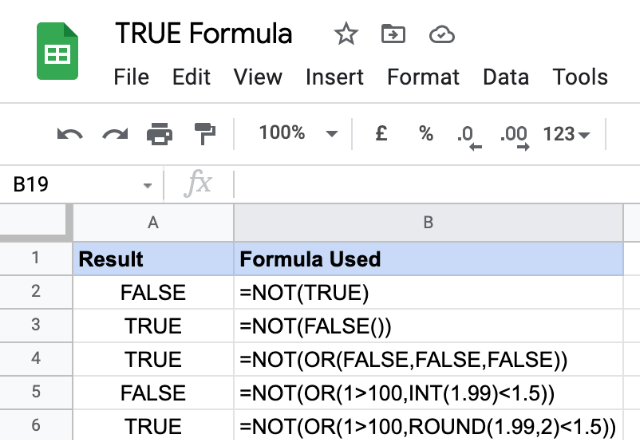# How to use the TRUE formula in Google SheetsThe TRUE formula in Google Sheets returns the logical value – TRUE. Perhaps, it is one of the most simplest and straightforward formulas.

### Syntax

TRUE()

Please note that the formula requires no input arguments.

In most of the scenarios, Google Sheets converts the literal text TRUE to the logical TRUE. So, to input the logical TRUE in a formula, we just have to key in the word TRUE directly and Google Sheets will interpret this as the logical TRUE.

Also, apart from using this formula directly to generate the logical TRUE value, the Google Sheets application can do this in many different ways. Some of the examples are shown in the snapshot below.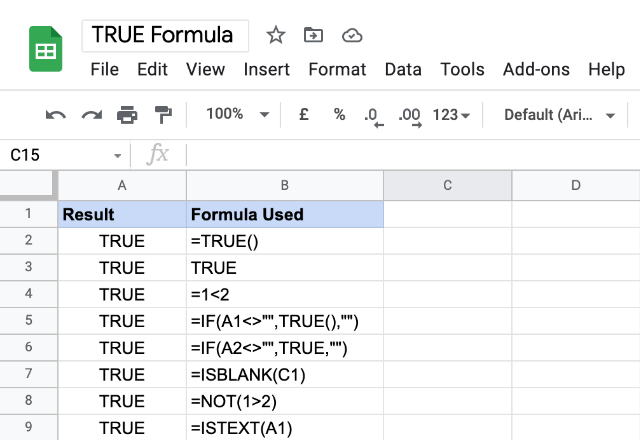### Usage: TRUE Formula

As a standalone formula, TRUE() may not have many practical uses. But, its presence is quite ubiquitous. Because the logical expressions that return the logical values form the cornerstones of many conditional formulas. Without the interplay between the logical expressions and the logical TRUE and FALSE values, many important formulas are as good as nothing. In the examples below, the conditional expressions enable the formulas to consider only the TRUE scenarios and ignore the ones that are FALSE.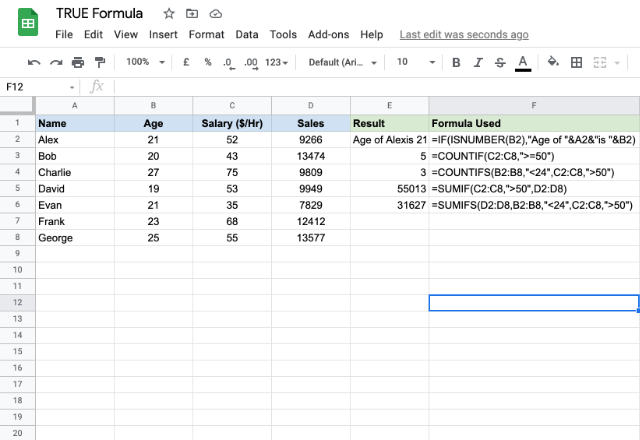##### TRUE Formula with AND, OR, and NOT

While we established that the logical TRUE and FALSE values are founding pillars on which many other formulas are build upon, we need to understand how the logical formulas AND, OR and NOT work with the logical TRUE and FALSE values.

##### AND Formula

This returns a logical TRUE if we set every input parameter value to logical TRUE. If we set any of the parameters to a logical FALSE, then, as a result, the output value is a logical FALSE.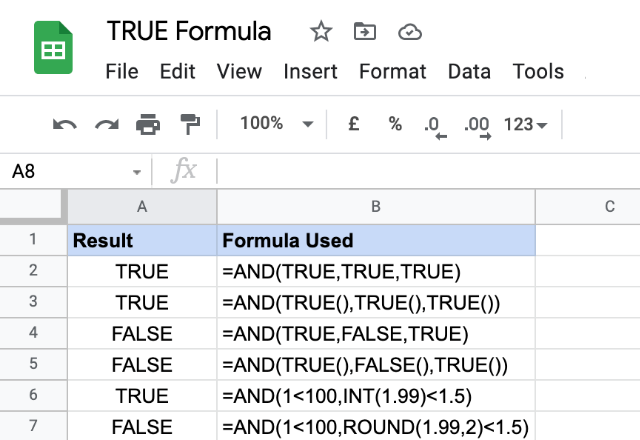##### OR Formula

This returns a logical TRUE if we set any of the input parameter values to logical TRUE. Therefore, if we set every input parameter to logical FALSE, then the output value is a logical FALSE.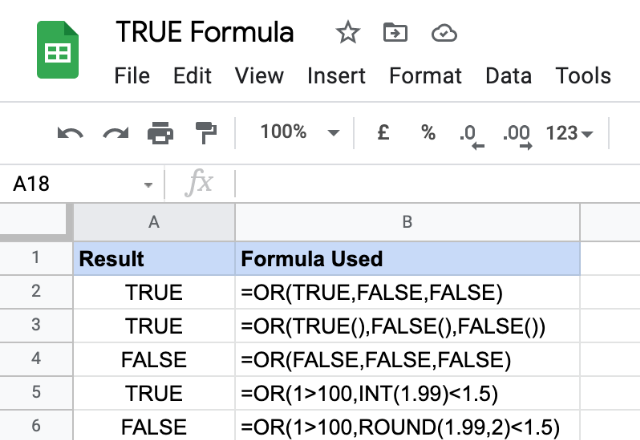##### NOT Formula

This returns a logical TRUE if we set the input parameter to logical FALSE, and vice versa. Hence, the output is just the negation of the input logical value.